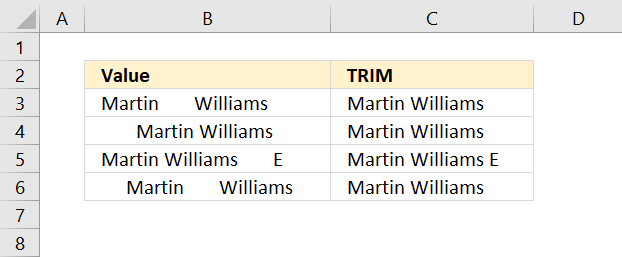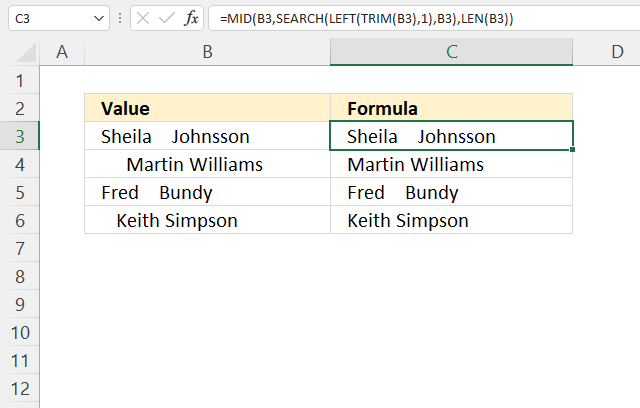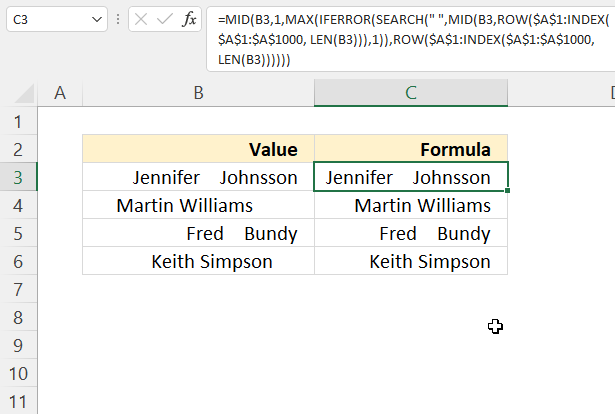Author: Oscar Cronquist Article last updated on May 17, 2022The picture above shows you values that have multiple leading and trailing blanks, sometimes also multiple blanks between words.

The TRIM function deletes all blanks or space characters except single blanks between words in a cell value.

Formula in cell C3:

=TRIM(B3)

TRIM(text)

## 2. TRIM Function Argument

 text The string or cell value you want to delete blanks from.

## 3. TRIM function not workingThe TRIM function removes the space character from a cell value, however, there is another space character commonly used in HTML pages that are not removed by this function.

To remove that space character you need to use the SUBSTITUTE function with the TRIM function. Formula in cell C3:

=TRIM(SUBSTITUTE(B3, CHAR(160), " "))

CHAR(160) returns the other space character that TRIM can't delete. The SUBSTITUTE function then replaces the HTML space character with a regular space character.

### 3.1 Explaining formula

#### Step 1 - Create HTML space character

The CHAR function converts a number to the corresponding character based on your computer's character set.

CHAR(160)

returns " ". This is an HTML space character.

#### Step 2 - Replace HTML character with a regular space character

The SUBSTITUTE function replaces a specific text string in a value.

SUBSTITUTE(text, old_text, new_text, [instance_num])

SUBSTITUTE(B3, CHAR(160), " ")

becomes

SUBSTITUTE("Martin       Williams"," "," ")

and returns

"Martin       Williams".

#### Step 3 - Remove leading and trailing space characters

TRIM(SUBSTITUTE(B3, CHAR(160), " "))

becomes

TRIM("Martin       Williams")

and returns "Martin Williams".

## 4. Remove leading space characters onlyFormula in cell C3:

=MID(B3, SEARCH(LEFT(TRIM(B3), 1), B3), LEN(B3))

### Explaining formula in cell C4

#### Step 1 - Remove leading, trailing, and repeated space characters

TRIM(B4)

becomes

TRIM(" Martin Williams ")

and returns

"Martin Williams"

#### Step 2 - Extract the first character

The LEFT function extracts a specific number of characters starting from the left.

LEFT(text, [num_chars])

LEFT(TRIM(B3),1)

becomes

LEFT("Martin Williams",1)

and returns "M".

#### Step 3 - Search for the first character

The SEARCH function returns a number representing the position of character at which a specific text string is found reading left to right.

SEARCH(find_text,within_text, [start_num])

SEARCH(LEFT(TRIM(B3),1),B3)

becomes

SEARCH("M", " Martin Williams ")

and returns 2.

#### Step 4 - Count characters

The LEN function returns the number of characters in a cell or string.

LEN(B3)

becomes

LEN(" Martin Williams ")

returns 21.

#### Step 5 - Extract string based on character position and string length

The MID function extracts a substring from a string based on the starting position and the number of characters you want to extract.

MID(textstart_numnum_chars)

MID(B3, SEARCH(LEFT(TRIM(B3), 1), B3), LEN(B3))

becomes

MID(B3, 2, 21)

becomes

MID(" Martin Williams ", 2, 21)

and returns "Martin Williams ".

## 5. Remove trailing space characters onlyThe values in columns B and C are right-aligned in order to show that there are multiple trailing space characters in cells B4 and B6.

Excel formula in cell C3:

=MID(B3, 1, MAX(IFERROR(SEARCH(" ", MID(B3, ROW(\$A\$1:INDEX(\$A\$1:\$A\$1000, LEN(B3))), 1)), ROW(\$A\$1:INDEX(\$A\$1:\$A\$1000, LEN(B3))))))

### Explaining formula in cell C4

#### Step 1 - Count characters

The LEN function returns the number of characters in a cell or string.

LEN(B3)

becomes

LEN("     Martin Williams      ")

and returns 29.

#### Step 2 - Create dynamic cell reference

The INDEX function is most often used to get values, however, it can also be used to create cell references.

INDEX(\$A\$1:\$A\$1000, LEN(B3))

becomes

INDEX(\$A\$1:\$A\$1000, 20)

and returns \$A\$20.

#### Step 3 - Create a cell reference to a cell range

The colon character lets you build cell references in an Excel formula.

\$A\$1:INDEX(\$A\$1:\$A\$1000, LEN(B3))

returns \$A\$1:\$A\$20.

#### Step 4 - Create numbers from 1 to n

The ROW function returns a number representing the row number of a given cell reference.

ROW(\$A\$1:INDEX(\$A\$1:\$A\$1000, LEN(B3)))

becomes

ROW(\$A\$1:\$A\$20)

and returns {1; 2; 3; 4; 5; 6; 7; 8; 9; 10; 11; 12; 13; 14; 15; 16; 17; 18; 19; 20}.

#### Step 5 -Extract string based on character position and string length

The MID function extracts a substring from a string based on the starting position and the number of characters you want to extract.

MID(textstart_numnum_chars)

MID(B3, ROW(\$A\$1:INDEX(\$A\$1:\$A\$1000, LEN(B3))), 1)

becomes

MID("     Martin Williams      ", {1; 2; 3; 4; 5; 6; 7; 8; 9; 10; 11; 12; 13; 14; 15; 16; 17; 18; 19; 20}, 1)

and returns

{" "; " "; " "; " "; " "; "M"; "a"; "r"; "t"; "i"; "n"; " "; "W"; "i"; "l"; "l"; "i"; "a"; "m"; "s"; " "; " "; " "; " "; " "; " "; " "; " "; " "}.

#### Step 6 - Search for blanks

The SEARCH function returns a number representing the position of character at which a specific text string is found reading left to right.

SEARCH(find_text,within_text, [start_num])

SEARCH(" ", MID(B3, ROW(\$A\$1:INDEX(\$A\$1:\$A\$1000, LEN(B3))), 1))

becomes

SEARCH(" ", {" "; " "; " "; " "; " "; "M"; "a"; "r"; "t"; "i"; "n"; " "; "W"; "i"; "l"; "l"; "i"; "a"; "m"; "s"; " "; " "; " "; " "; " "; " "; " "; " "; " "})

and returns {1; 1; 1; 1; 1; #VALUE!; #VALUE!; #VALUE!; #VALUE!; #VALUE!; #VALUE!; 1; #VALUE!; #VALUE!; #VALUE!; #VALUE!; #VALUE!; #VALUE!; #VALUE!; #VALUE!; 1; 1; 1; 1; 1; 1; 1; 1; 1}.

#### Step 7 - Replae errors with corresponding number

The IFERROR function handles error values.

IFERROR(valuevalue_if_error)

IFERROR(SEARCH(" ", MID(B3, ROW(\$A\$1:INDEX(\$A\$1:\$A\$1000, LEN(B3))), 1)), ROW(\$A\$1:INDEX(\$A\$1:\$A\$1000, LEN(B3))))

becomes

IFERROR({1; 1; 1; 1; 1; #VALUE!; #VALUE!; #VALUE!; #VALUE!; #VALUE!; #VALUE!; 1; #VALUE!; #VALUE!; #VALUE!; #VALUE!; #VALUE!; #VALUE!; #VALUE!; #VALUE!; 1; 1; 1; 1; 1; 1; 1; 1; 1}, ROW(\$A\$1:INDEX(\$A\$1:\$A\$1000, LEN(B3))))

becomes

IFERROR({1; 1; 1; 1; 1; #VALUE!; #VALUE!; #VALUE!; #VALUE!; #VALUE!; #VALUE!; 1; #VALUE!; #VALUE!; #VALUE!; #VALUE!; #VALUE!; #VALUE!; #VALUE!; #VALUE!; 1; 1; 1; 1; 1; 1; 1; 1; 1}, {1; 2; 3; 4; 5; 6; 7; 8; 9; 10; 11; 12; 13; 14; 15; 16; 17; 18; 19; 20})

and returns

{1; 1; 1; 1; 1; 6; 7; 8; 9; 10; 11; 1; 13; 14; 15; 16; 17; 18; 19; 20; 1; 1; 1; 1; 1; 1; 1; 1; 1}.

#### Step 8 - Calculate the largest number in the array

The MAX function returns the largest number in an array or cell range.

MAX(number1, [number2], ...)

MAX(IFERROR(SEARCH(" ", MID(B3, ROW(\$A\$1:INDEX(\$A\$1:\$A\$1000, LEN(B3))), 1)), ROW(\$A\$1:INDEX(\$A\$1:\$A\$1000, LEN(B3)))))

becomes

MAX({1; 1; 1; 1; 1; 6; 7; 8; 9; 10; 11; 1; 13; 14; 15; 16; 17; 18; 19; 20; 1; 1; 1; 1; 1; 1; 1; 1; 1})

and returns 20.

#### Step 9 - Extract string from value

The MID function extracts a substring from a string based on the starting position and the number of characters you want to extract.

MID(textstart_numnum_chars)

MID(B3, 1, MAX(IFERROR(SEARCH(" ", MID(B3, ROW(\$A\$1:INDEX(\$A\$1:\$A\$1000, LEN(B3))), 1)), ROW(\$A\$1:INDEX(\$A\$1:\$A\$1000, LEN(B3))))))

becomes

MID("     Martin Williams     ", 1, 20)

and returns "     Martin Williams".

### Excel 365 formula

Excel 365 formula in cell C3:

=LET(x, B3, y, SEQUENCE(, LEN(x)), MID(x, 1, MAX(IFERROR(SEARCH(" ", MID(x, y, 1)), y))))